# Acta Universitatis Danubius. Œconomica, Vol 10, No 5 (2014)

A Comparative Analysis of Some Results from Qp and R

Alin Cristian Ioan1

Abstract: The paper investigates whether a series of concepts and properties available in the real analysis remains valid for p-adic case. There are many similarities between R and Qp and also so many differences. First of all, R is an ordered field, which is not true for Qp. Secondly R is archimedean (that is the absolute valuationis archimedean) while Qp is not archimedean for any p prime. This means that R is a connected metric space while Qp is totally disconnected. This proves that there is no analogous notion of interval in Qp or a notion similar to the curve. These contrasts will cause the difference between the analysis p-adic and the real analysis.

JEL Classification: C02

1 Introduction

Let note Qp the field of p-adic numbers. Before we begin, we should note that there are many similarities between R and Qp and also so many differences. First of all, R is an ordered field, which is not true for Qp. Secondly R is archimedean (that is the absolute valuationis archimedean) while Qp is not archimedean for any p prime. This means that R is a connected metric space while Qp is totally disconnected. This proves that there is no analogous notion of interval in Qp or a notion similar to the curve. These contrasts will cause the difference between the analysis p-adic and the real analysis.

2 Sequences and Series in Qp

We begin by studying the basic properties of strings and series in Qp. The most important thing about Qp is that the field is a complete field, therefore every Cauchy sequence is convergent. Naturally all the properties of the norm 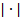 on R are the same of the properties of the p-adic valuations (the property of being non-archimedean being an additional property).

As a result, many of the basic theorems that occur in the real analysis, taking place also in the p-adic analysis. One of the great benefits of the p-adic analysis is that it will bring generalizations to some real questions raised in the analysis (due to the property of p to be non-archimedean).

Lemma 1

A sequence (xn)Qp is a Cauchy sequence if and only if 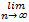 |xn+1 – xn| = 0.

Proof

If m=n+r>n, we get | xm – xn| = | xn+r – xn+r-1 + xn+r-1 - xn+r-2 +...- xn| max { | xn+r – xn+r-1 |,
| x
n+r-1 - xn+r-2 |,..., |xn+1 – xn| } this fact being true because p is non-archimedean. Now for r N* and > 0 N N* such that |xm – xn| = |xn+r – xn| max { | xn+r – xn+r-1 |, | xn+r-1 - xn+r-2 |, ...,|xn+1 – xn| } < n, m N. N is that natural number which n N we have |xn+1 – xn | < . Therefore, the sequence (xn) Qp is Cauchy so convergent.

The theory of sequences and their convergence is therefore similar with that on R except lemma above.

Proposition 2

Let (an ) Qp a convergent sequence. Then we have one of two statements: either lim |an| = 0, or there exists an integer M such that |an| = |aM| n M. In other words, the absolute value of the sequence converges to zero or it becomes constant after a rank on.

Proof

Suppose that lim |an| 0 > 0 such that N1 N*, n N1 with |an| . So a number c > > 0 with |an| c > , n N1. On the other hand N2 integer for which n,m N2 | an - am| < c. We want both conditions occur so fix > 0 N = max { N1, N2}. Now n,m N | an - am| < max { |an|, |am| } from where we get |an| = |am| after non-archimedean property (that is, in the space Qp all triangles are isosceles).

Also, for series the classical theory remains valid. For example, the following statements are true:

Proposition 3

Let (an) Qp. The absolute convergence of sequence implies its convergence, ie if a series of absolute values 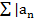| converges in R then the series 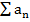 converges in Qp.

Proof

The series  converges in Qp 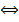 lim |an| = 0. But a necessary condition for absolute series to converges is that lim |an| = 0.

The next result is a strong result in real analysis, but in p-adic context, the previous lemma becomes an important tool to determine whether a series of p-adic numbers converges in Qp namely:

Corollary 4

An infinite series 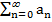 with (an) Qp is convergent   an = 0. In this case we also have |  | 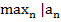|.

Proof

A series converges only when the sequence of partial sums converges. Now take the difference between the n-th partial sum and the (n-1)-th. By Lemma we get that this difference tends to 0 as we wanted. Conversely we have the sequence of partial sums is Cauchy therefore convergent. If =0 we have nothing to prove. Otherwise, for any partial sum, we have | 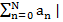 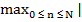an|. Since  an = 0 > 0 N N* such that |an| < n > N = N. Let = an|. Thus we have an| = 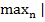an|. How an| does not depend on N for N 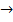 we get |  | |, that is the conclusion.

The reciprocal question related to when a series is convergent in R implies that its general term tends to zero is not necessarily true. As a counterexample we have the harmonic series which not converges in R.

Therefore, it is much easier to establish convergence of the infinite series in p-adic context than in R. This seems to express that the theory of series in Qp is much simpler than in R.

Now we shall consider a “double string” (bij) Qp asking what happens to the two series considered after a summing with i and after j or viceversa. For this, it is necessary that, as example, bij0 when one of the indices is fixed and the other goes to infinity (otherwise obvious series will not converges). We shall say that 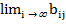 = 0 uniformly in j if > 0 we can find an integer N which does not depend on j such that i N | bij | < j. In other words, the sequence (bij) tends to 0 when i  , the convergence coming from the same rank for all j. First we prove the following lemma:

Lemma 5

Let (bij) Qp and assume that:

1 ) i, 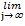 bij = 0

2) 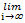 bij = 0 uniformly in j

Then for any real number > 0 an integer N which depends only of such that if max(i, j) N |bij| < .

Proof

Let > 0 fixed. The second condition says that we can choose N0 N*, which depends on but not of j such that |bij| < if i N0. The first condition is weaker (it says basically that i we can find N1(i ) N*, “the notation suggesting that the whole depends on i”) such that if j N1(i ) we have |bij| < . Now we take N = N() = max (N0, N1(0), N1(1), …, N1(N0 – 1)). The choice of N was done so that if max (i,j ) N then i N0 when |bij| < regardless of j or if i < N0 j N and i { 0,1,2,…, N0 – 1} therefore j N1(i ), when we have |bij| < .

Proposition 6

Let (bij) Qp and assume that:

1. i,  bij = 0

2.  bij = 0 uniformly in j

Then the series 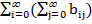 and 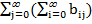 converges and their sums are equal.

Proof

From the previous lemma we know that for a given > 0 we can choose N such that for max (i, j) N |bij| < . In particular for i, when j  or viceversa then the inner sums 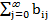 and 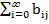 converges (the first sum for each i and the second for each j). More, for i N we have | | 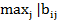| < . Similarly for any j N we have | | < . In particular, we note that 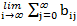 = 0 and 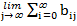 = 0 therefore both series converges. It remains to show that the sums of the two double series are equal. We will continue to use N and as above so that the condition |bij| < i or j N holds. We will often use the ultrametric inequality: |x + y| max{ |x|, |y|} applied even at the level of series as we have seen in the last corollary. We see first |  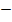 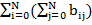| = | 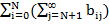  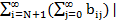. Now for j N+1 we shall have |bij| < i. With ultrametric inequality it remains that | 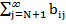| < i and, using again the ultrametric inequality we have that | | < . Similarly, we obtain | < . So, again applying this inequality we have that: |   | < . Reversing now i with j we get a similar inequality that is |   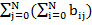| < , then finally
|
  | < . But how was arbitrarily fixed the double series are equal.

What basically says this proposition is that if the double sequence {bij} converges to 0 in a uniform way, then the double sum after i and j can be taken in any order to give the same answer.

Now if a = 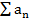 and b = 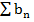are two convergent series, then the series + bn is convergent and has the sum a + b. Indeed, the first sum is convergent  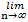 an = 0 and so the second if  bn = 0. In conclusion,  an + bn = 0, which is enough to say that the series + bn converges. Now, noting with c the sum of the series we have that 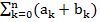 = 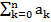 + 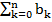 and passing to the limit with n  we have that c = a + b.

A second problem is related in some way to the top as follows: if a =  and b =  are two convergent series, taking cn = bn-k then the series 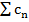 is convergent and its sum is ab.

Let the partial sum of order n of a and the partial sum of order n of b that is sn =  and tn = 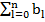. Now sntn = 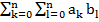. As above, we have:  an = 0 and  bn = 0. Computing 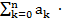  - 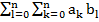 for n N. In short, this expression is written sntn - cn - 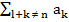bl = 0 where l and k go through the set of numbers 0,...,n. Finally, we have: sntn – cn - cn-1 - cn-2 +...- c0 – cn+1 -... – c2n = 0 ie passing to the limit with n  we get ab = 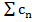 that is c = ab.

3 Functions, Continuity, Differentiability in Qp

The basic idea on the functions and continuity remains unchanged by the passage of real numbers to p-adic numbers because ultimately they depend on the metric structure. Not be able to work with intervals (nay nor related with nontrivial connected sets), so that our functions will be defined on disks (closed-open). We shall write B(a,r) for open sets of center a and radius r> 0 and 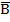(a,r) for the closed sets of center and radius r.

Definition 7

Let U Qp be an open set. A function f: U  Qp is called continous in a U if > 0  > 0 such that x U with the property | x – a| < | f(x) – f(a)| < .

The base results on continuity are true in all metric spaces and therefore also in the p-adic fields. For example, if U is a compact set (and remember that Qp is both open and compact so a subset included in it can have these properties) and f is continuous at any point in U then f is uniformly continuous. Automaticaly, the Darboux property to carry an interval within an interval is true since the intervals in Qp are identified with points. In the general context, the Darboux property says that a continuous function defined on a metric space carry a connected set into another connected set.

Now, if U = Zp then for any a Zp, > 0, n N with x Zp such that | x – a | < 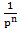, we have | f(x) – f(a)| < . However = 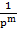 for m Z. For m = 0 we have that f(x) – f(a) Zp that is f(x) is in one of the neighbourhoods (closed-open) of f(a) ie f carry a local connected set into a local connected set.

Derivatives are perhaps more interesting from the fact that there is a lower analogy with the classical real case. It will make sense to define derivatives of functions f: Qp  Qp in the usual way, namely:

Definition 8

Let U Qp be an open set and let f: U  Qp a function. We say that f is differentiable in xU if the limit f(x) = 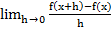. If f (x) exists for any x U we shall say that f is differentiable on U and we write: f: U  Qp for the function x  f (x).

Remark

Up to a certain point, the derivative of a function with values in Qp behaves as if real, that is it can be shown that a differentiable function is continuous as shown in R or C.

It is natural to ask what is the role of the derivative of a function in the p-adic case. But if we consider that the mean value theorem states for a and b real data in the domain of definition of a differentiable function (while continuing) between a and b such that f (b) - f (a ) = f '() (b - a), is not working in the p-adic case, because in fact we have not the relation of “being between” because Qp is not an ordered field. But this slight inconvenience can be simply remedied if we think that in R we can define the relation “being between” saying that is between a and b if we have =at+b(1-t) for 0 t 1. Nearly the same happens in the complex case. What we can now express through the mean value theorem in the p-adic case? We ask if there the statement holds: if we have a function f defined on Qp, differentiable and continuous on Qp then for any two numbers a and b in Qp Qp of the form: =at+b(1-t) for t such that |t| 1, for which f(b) – f(a)=f () (b – a). We shall show that the mean value theorem for p-adic case is false.

Proof

Let f(x) = xp – x, a = 0, b = 1. We have f (x) = pxp-1 – 1 and f(a) = f(b) = 0. If the statement is true, it exists Qp of the form = at + b(1 - t) = 1 – t with t Zp such that pp-1 – 1 = 0. But from here and Zp and from pp-1 – 1 = 0 0 1 + p Zp - contradiction.

4 References

Gouvea, Fernando Q. (1997). P-adic numbers. An introduction. 2nd Edition. New York, Heidelberg, Berlin: Springer-Verlag.

Ioan, A.C. (2013). Through the maze of algebraic theory of numbers. Galati: Zigotto Publishing.,

Ireland, Kenneth & Rosen Michael (1990). A classical introduction to modern number theory, Springer.

Marcus, Daniel (1977). Number fields. New York: Springer Verlag.

Neukirch, Jurgen (1999). Algebraic number theory. New York, Heidelberg, Berlin: Springer Verlag.

Roquette, Peter (2003). History of valuation theory. Part I, Heidelberg

1 University of Bucharest, Faculty of Mathematics and Computer Science, Romania, Address: 4-12 Regina Elisabeta Blvd, Bucharest 030018, Romania, Corresponding author: alincristianioan@yahoo.com.

AUDŒ, Vol. 10, no. 5, pp. 52-58

### Refbacks

• There are currently no refbacks.